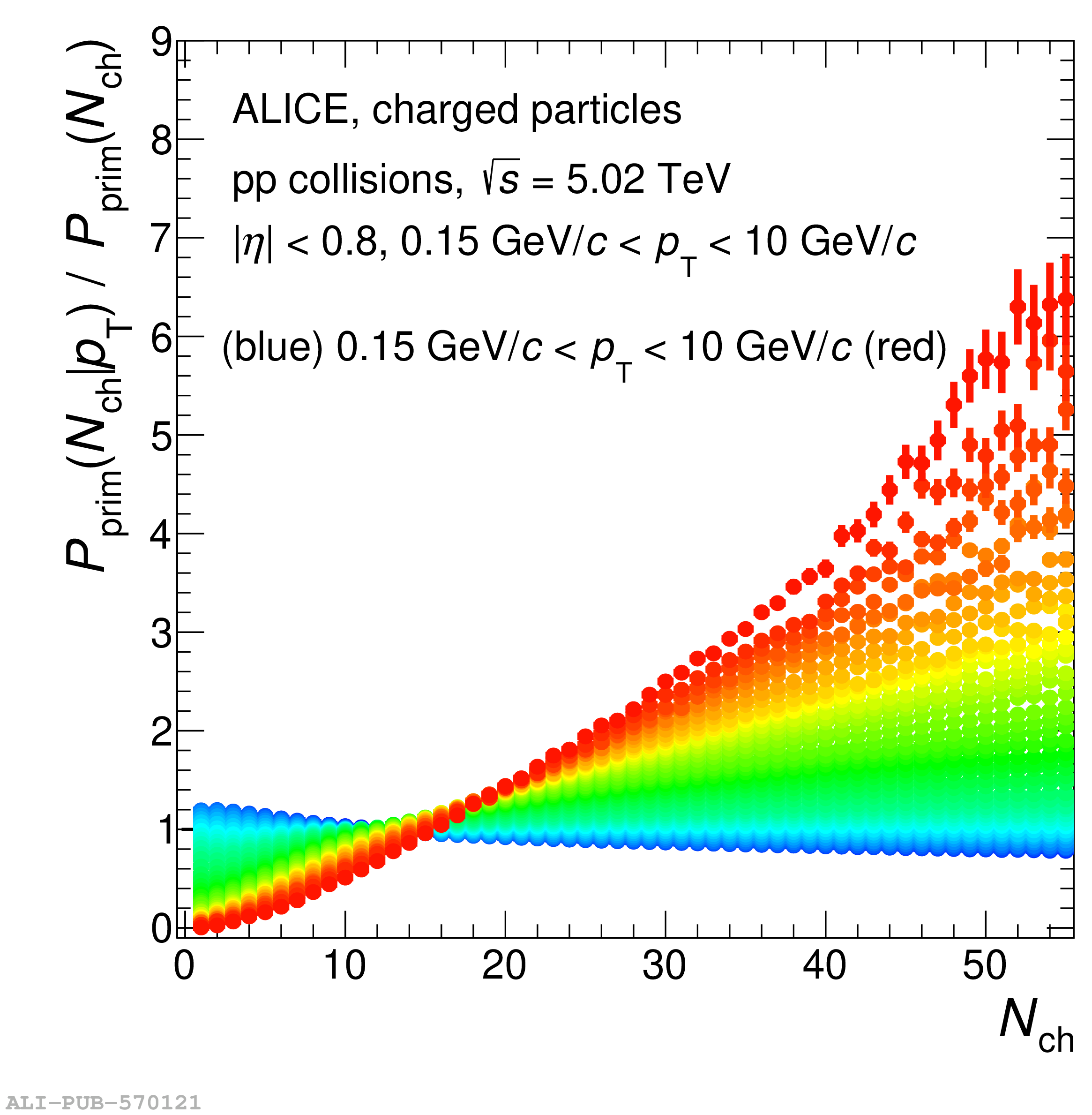# Figure 2

 Top panel: the correlation of primary charged particle \pt spectra with multiplicity per $\nch > 0$ event for \pp collisions at $\sqrt{s} = 5.02\tev$ Bottom panels: the corresponding relative change of \pt (left) and \nch (right) distributions with respect to the inclusive ones. In the left panel, each of the curves represents a single \nch value, ranging from $\nch = 1$ (blue) to $\nch = 55$ (red). In the right panel, the colours represent the \pt intervals used in this analysis from the lowest in blue to the highest one in red.